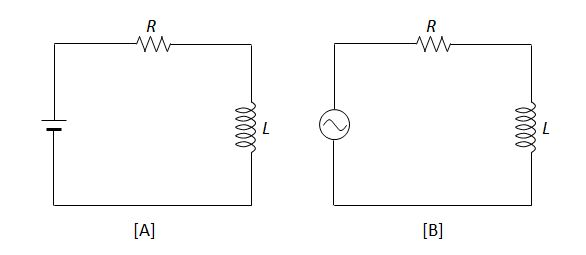# RL circuitThe above are RL series circuits, where the resistance of the resistor and the self-inductance of the inductor are $R$ and $L$, respectively. The voltage of the DC power supply(A) is the same as the RMS(root mean square) value of the AC voltage(B). Which of the following statements is correct?

a) The current intensity flowing in circuit A is greater than the RMS current intensity flowing in circuit B.

b) The greater the resistance R, the smaller the current intensity flowing in the circuit, for both A and B.

c) The greater the inductance L of circuit B, the greater the current intensity flowing in circuit B.

×